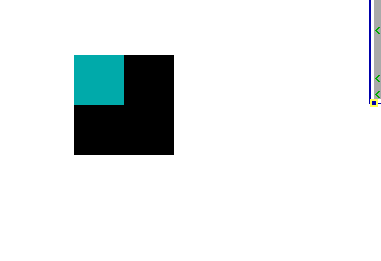# Fun with Graphics

Graphics are the things your user see's,and TempleOS can do much more than just text. Graphics are done via `CDC`'s also known as Drawing Contexts. Each window has a function pointer in `Fs->draw_it` which points to drawing function. Let's see what it looks like.``//The first argument is the CTask being fdrawU0 DrawIt(CTask *,CDC *dc) {  dc->color=BLUE;  GrRect(dc,0,0,100,100);}Fs->draw_it=&DrawIt;``

Here we will use a function pointer to draw the screen.
The function used here takes 2 arguments,the `CTask`and the `CDC`.
The `CDC` is a drawing context,and we use functions to draw on it like `GrRect`.
We can also edit the dc directly to change the color of the drawer.

There are other Graphics functions as well...

``U0 DrawIt(CTask *,CDC *dc) {  dc->color=BLUE;  GrLine(dc,0,0,100,100);  GrCircle(dc,100,100,50);  dc->color=RED;  GrFillCircle(dc,0,0,,50);  GrPlot(dc,100,100); //Single point  GrRect(dc,200,200,100,100);  dc->color=YELLOW;  GrPrint(dc,0,0,"Hello %s","World");}Fs->draw_it=&DrawIt;``## Drawing Contexts​

Drawing contexts are what let us actually draw to the screen.
We can create drawing contexts with `DCNew(w,h)`. These aren't draw to the Screen, but you can blot them to the screen via `GrBlot`

``CDC *new=DCNew(100,100);DCClear(new);//Clear our new CDCnew->color=CYAN;GrRect(new,0,0,50,50);U0 DrawIt(CTask *,CDC *dc){  GrBlot(dc,100,100,new);}Fs->draw_it=&DrawIt;``We can access the same image in 2 different drawing contexts,we can do this via `DCAlias`
`DCAlias` will make a copy of a CDC,but it will point to the same image in RAM.
Here's an Example:

`` CDC *old=DCNew(100,100); DCClear(old); //Clear our new CDC old->color=CYAN; GrRect(old,0,0,50,50); CDC *new=DCAlias(old); //We are going to modify old's contents through new new->color=YELLOW; GrRect(new,50,50,50,50); GrBlot(NULL,0,0,old); //old was modified by new Sleep(1000); DCFill; DCDel(old); //Free our CDC's DCDel(new);``## Transformations​

TempleOS uses transformation matrices to do things like rotation and scaling and other 3D stuff. These transformations can be combined. Here,we rotate a circle around the center by using 2 transformations here,a Z rotation,and a translation transformation. The Z rotation is good for 2D transformations,and we set the center of the rotation to 50,50 via Mat4x4TranslationEqu. It looks silly and epic. We must also enable transformations via DCF_TRANSFORMATION,( and use a graphics function typically ending in "3")

``CDC *dc=DCNew(100,100); dc->r=Mat4x4IdentNew; DCFill; dc->flags|=DCF_TRANSFORMATION; F64 rotx=0; for(;rotx<=2*pi;rotx+=(2*pi/100.)) {        DCFill(dc);         Mat4x4IdentEqu(dc->r); //Reset our transformation         Mat4x4RotZ(dc->r,rotx);         Mat4x4TranslationEqu(dc->r,50,50,0);          dc->color=YELLOW;         GrRect3(dc,0,0,0,50,50);         DCFill;         GrBlot(,100,100,dc);         Sleep(33); } DCDel(dc); DCFill;``Try switching up the X_Y_Z rotations.

• The Z axis points towards the screen.
• The X axis points to the left.
• The Y axis points up.

## Transformations(2)​

Transformations use a matrix,which I wont explain the mathematics in much detail here.
There is a thing called the identity matrix,which is the "default" matrix.
To reset the drawing context's matrix,do this

``Mat4x4IdentEqu(dc->R);// Or if you want a new Matrixdc->R=Mat4x4IdentNew;``

We can combine transformations on a single matrix,be sure to do them in the order you want

`` CDC *dc=DCNew(200,200); dc->r=Mat4x4IdentNew; DCFill; dc->flags|=DCF_TRANSFORMATION; F64 rotx=0; for(;rotx<=2*pi;rotx+=(2*pi/100.)) {         DCFill(dc);         Mat4x4IdentEqu(dc->r);         Mat4x4RotX(dc->r,rotx);         Mat4x4RotY(dc->r,rotx);         Mat4x4RotZ(dc->r,rotx);         //Be sure to translate to the center         Mat4x4TranslationEqu(dc->r,50,50,0);         Mat4x4Scale(dc->r,2.);         dc->color=YELLOW;         GrRect3(dc,0,0,0,50,50);         DCFill;         GrBlot(,100,100,dc);         Sleep(33); } DCDel(dc); DCFill;``

We can use depth buffer,now for a cube without the top and bottom

• Use DCDepthBufAlloc to allocate a depth buffer
• Be sure to DCDepthBufRst after each call!!!.
• The z buffer is symbolic and is only used for checking if an item is behind another. It doesn't shrink at stuff goes farther away.
`` CD3I32 poly= {{-100,-100,-100},{100,-100,-100},{100,100,-100},{-100,100,-100}}; I64 colors= {BLUE,YELLOW,GREEN,CYAN}; CDC *dc=DCNew(200,200); dc->r=Mat4x4IdentNew; DCDepthBufAlloc(dc); DCFill; dc->flags|=DCF_TRANSFORMATION; F64 rotx=0,roty; CD3I32 cube; I64 i=0,i2=0; I64 *trans=Mat4x4IdentNew; for(rotx=0.; rotx<=(2.*pi)-1.; rotx+=2*pi/4.) {     //Mat4x4TranslationEqu(trans,50,50,50);     Mat4x4IdentEqu(trans);     Mat4x4RotX(trans,rotx);     Mat4x4RotY(trans,roty);     for(i2=0; i2!=4; i2++) {         MemCpy(&cube[i][i2],&poly[i2],sizeof(CD3I32));         Mat4x4MulXYZ(trans,&cube[i][i2].x,&cube[i][i2].y,&cube[i][i2].z);     }     i++; } for(rotx=0; rotx<=2*pi; rotx+=(2*pi/100.)) {     DCFill(dc);     DCDepthBufRst(dc);     Mat4x4IdentEqu(dc->r);     Mat4x4RotX(dc->r,rotx);     Mat4x4RotY(dc->r,rotx);     Mat4x4RotZ(dc->r,rotx);     Mat4x4Scale(dc->r,.5);     Mat4x4TranslationEqu(dc->r,0,0,3000);     for(i2=0; i2!=6; i2++) {         dc->color=colors[i2];         GrFillPoly3(dc,4,cube[i2]);     }     DCFill;     GrBlot(,100,100,dc);     Sleep(33); } DCDel(dc); DCFill;``Eariler I said that Z doesnt shrink as you go farther,that's because we need a transformation callback!
We can put such a callback in `CDC.transform` with the `DCF_TRANSFORMATION` flag. We divide by z to get a simulate going back.
Let's make a shrinking rectangle

`` #define SCRN_SCALE 512 U0 Transform(CDC *dc,I64 *x,I64 *y,I64 *z) {   I64 zz;   Mat4x4MulXYZ(dc->r,x,y,z);   zz=SCRN_SCALE/3+*z;   if (zz<1) zz=1;   *x=SCRN_SCALE/2* *x/zz;   *y=SCRN_SCALE/2* (*y)/zz;   *x+=dc->x;   *y+=dc->y;   *z+=dc->z; } CDC *dc=DCAlias; dc->transform=&Transform; dc->flags|=DCF_TRANSFORMATION; I64 dist=0; dc->z=-60; for(dist=0;dist!=100;dist++) {     Mat4x4TranslationEqu(dc->r,0,0,dist);     dc->color=LTRED;     GrRect3(dc,0,0,0,100,100);     Refresh;     Sleep(3);     DCFill; }``

## Super Color Dithering​

Colors can be combined via dithering via `ROPF_DITHER`. Let's see an example.

`` CDC *dc=DCAlias; I64 cnt; for(cnt=0;cnt!=100;cnt++) {     dc->color=LTRED+YELLOW<<16+ROPF_DITHER;     GrRect3(dc,0,0,0,100,100);     Refresh;     DCFill; }``

We can do a sort of 3d shading with `ROPF_PROBABILITY_DITHER`(it get weird with primitive operations,so use `GrFloodFill`)

`` CDC *dc=DCAlias; I64 cnt; for(cnt=0;cnt!=100;cnt++) {     dc->color=BLACK;     GrRect(dc,0,0,100,100);     dc->color=LTRED+YELLOW<<16+ROPF_PROBABILITY_DITHER;     dc->dither_probability_u16=U16_MAX*ToF64(cnt)/100.;     GrFloodFill(dc,10,10);     Refresh;     DCFill; } DCDel(dc);``